Next: Non-deterministic Finite Automata with instantaneous Up: Finite Automata Previous: Deterministic Finite Automata

## Non-deterministic Finite Automata

NON-DETERMINISTIC FINITE AUTOMATA. A non-deterministic finite automaton (NFA) consists of five things:

• an input alphabet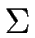,
• a finite set S whose elements are called states,
• a set I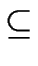S of distinguished states, called initial states,
• a set FS of distinguished states, called accepting states,
• a function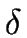from S×to 2S, thus every state-symbol couple is mapped byto set of states (that is a subset of S).
Observe that
• In the transition graph of a NFA the same symbol a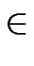can label two or more transitions out of one state.
• Therefore after reading a given symbol, a NFA can have several states.

LANGUAGE RECOGNIZED BY A NFA. A word w is accepted by a NFA if after reading w the NFA is in at least one accepting state. Figure 5 shows a NFA and a DFA recognizing the same language: the language over= {a, b} consisting of the words which end with b.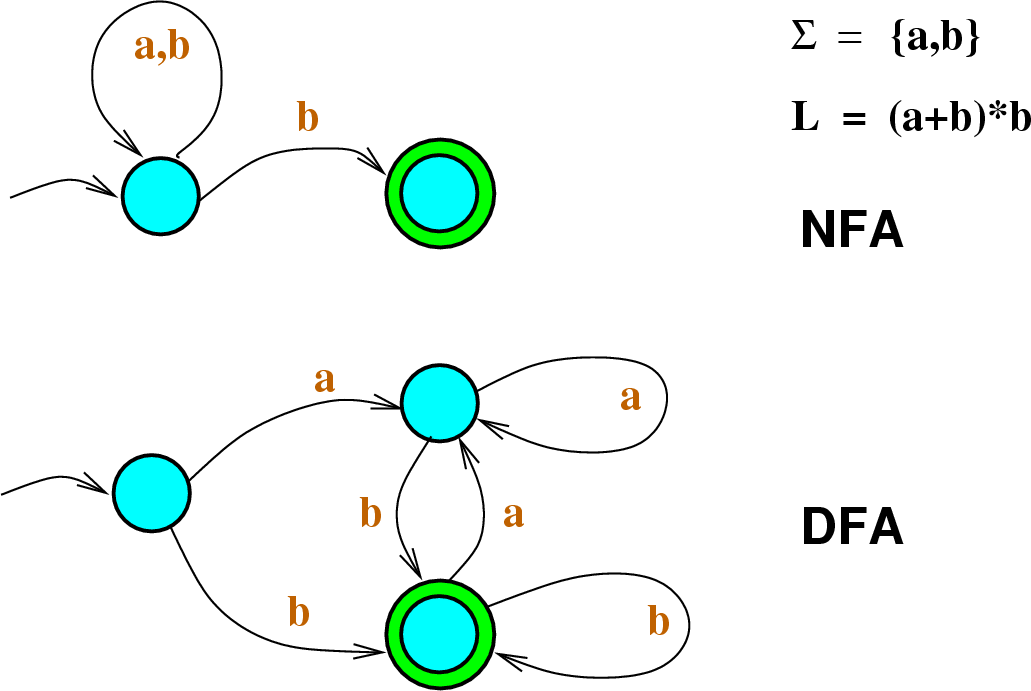FROM A NFA TO A DFA ACCEPTING THE SAME LANGUAGE.

Theorem 1   Let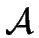= (, S, I, F,) be a NFA accepting the language L. Then there exists a DFA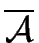accepting L too.

To construct such a DFAfromone proceeds as follows.

1. The alphabet is unchanged.
2. The set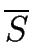of states ofis a subset of 2S. Hence each state ofis a subset of S. The setis computed by Algorithm 1 below.
3. The starting state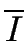ofis the set I of the initial states of.
4. The set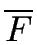of the accepting states ofis the set of the states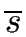ofsuch that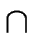F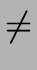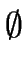.
5. The transition function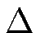oftogether withis determined by Algorithm 1, called the SUBSET ALGORITHM.

Algorithm 1
1
toSee := [];
2:= [ ];
3
while toSee[ ] repeat
3.1
A := first(toSee); toSee := rest(toSee);
3.2
for xrepeat
3.2.1(A, x) := {s' | (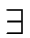sA) (s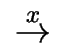s')};
3.2.2
if ((A, x)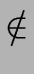toSee) and ((A, x)) then toSee := cons((A, x), toSee);
3.3:= cons( A,);
4
return(,)

In this algorithm
• toSee is the list of the statesofsuch that the(a,) for all ahave not been computed yet,
• whereascontains those statesoffor which(a,) is known for every a.
• the functions first, rest and cons operate on lists and are similar to the traditional stack routines top, pop and push respectively.
Figure 6 shows a DFA obtained from a NFA by applying Algorithm 1.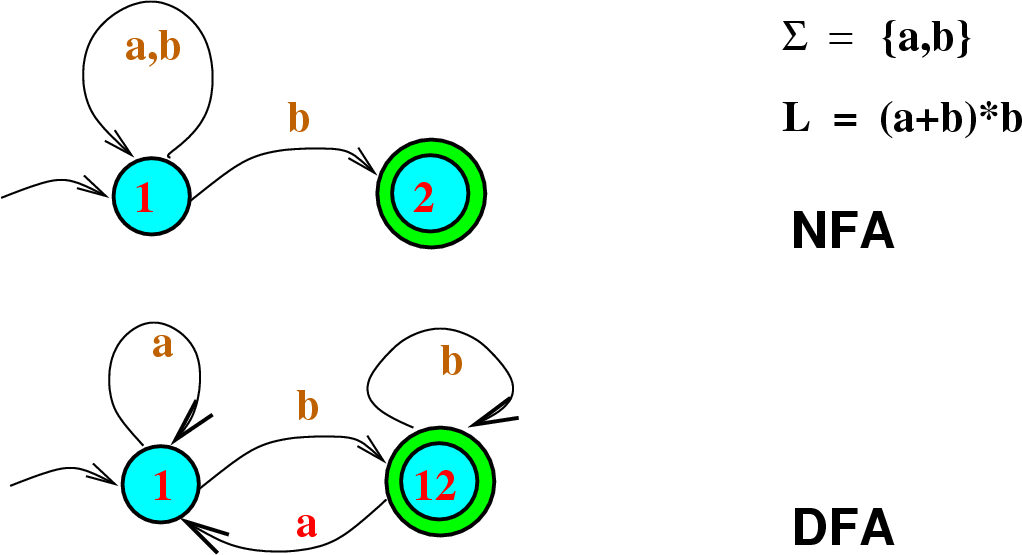Remark 1   States are generally denoted with numbers. So if the states of the input NFA are called 1, 2, 3,... then the states of the output DFA could be {1}, {2}, {3}, {1, 2}, {1, 3}, {2, 3}, {1, 2, 3}, .... To simplify notation we denote the subset {i, j,...}, by ij ... providing that there is no ambiguity.Next: Non-deterministic Finite Automata with instantaneous Up: Finite Automata Previous: Deterministic Finite Automata
Marc Moreno Maza
2004-12-02